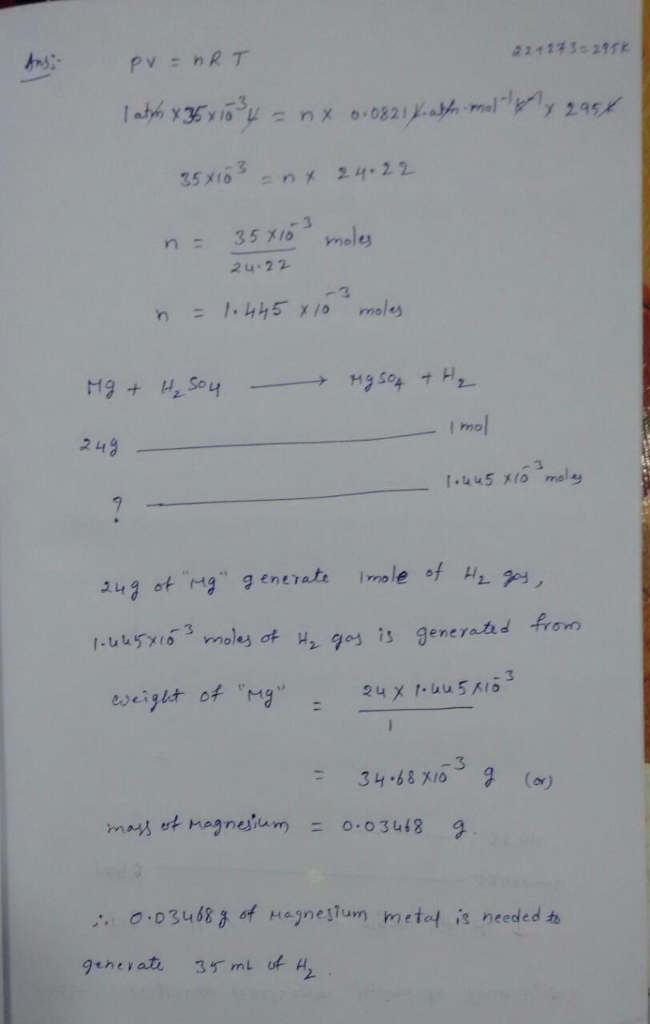# Homework Solution: Calculate the mass of magnesium metal needed to generate 35.0 mL of H2 gas at a pressure of…

Calculate the mass of magnesium metal needed to generate 35.0 mL of H2 gas at a pressure of 1.00 atm and a temperature of 22.0 °C HINT: Calculate the moles of H2 gas first (assuming ideal behavior), then use the equation above and stoichiometry to calculate the mass of Mg needed.